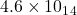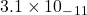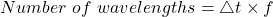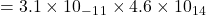## A certain type of laser emits light that has a frequency of 4.6 x 1014 Hz. The light, however, occurs as a series of short pulses, each last

Question

A certain type of laser emits light that has a frequency of 4.6 x 1014 Hz. The light, however, occurs as a series of short pulses, each lasting for a time of 3.1 x 10-11s. The light enters a pool of water. The frequency of the light remains the same, but the speed of light slows down to 2.3 x 108 m/s. In the water, how many wavelengths are in one pulse

in progress 0
4 weeks 2021-08-31T14:11:24+00:00 1 Answers 0 views 0

14,260

Explanation:

Relevant data provided for computing the wavelengths are in one pulse is here below:-

The number of wavelengths in Ls =Therefore the Number of in time = Δt =The number of wavelengths are in one pulse is shown below:-= 14,260

Therefore for computing the number of wavelengths are in one pulse we simply applied the above formula.